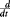# Hill's Equation of Muscle Contraction

The following article is from The Great Soviet Encyclopedia (1979). It might be outdated or ideologically biased.

## Hill’s Equation of Muscle Contraction

an equation that expresses the change in the speed of a muscle contraction as a function of load. It was derived by the British physiologist A. V. Hill in 1938.

Hill’s equation is expressed as (P + a)(v + b) = b (P0 + a) where v is the speed of a muscle contraction under a load P, P0 is the maximum value of the isometric force during tetanic stimulation of the entire muscle, and constants a and b are empirical values. The constant a has the dimensionality of force and is equal to about 4 × 105 dynes/cm2 of a cross section of various types of muscles, while the constant b has the dimensionality of velocity, expressed in cm/sec or l0/sec, where l0 is the initial length of the muscle; the latter constant differs for various muscles.

This regularity was expressed in more general form in 1953 by the British scientists B. C. Abbott and D. R. Wilkie. If the contracting muscle has a length l at the moment t, then the velocity of its contractiion dl/dt is determined by the formula – dl/dt = (F1F)b/(F + a) where F is the force that overcomes the muscle, F1 is the maximum force of the muscle at the length at which the velocity of its contraction is measured, and a and b are constants. This formula was modifed by Wilkie in 1956, which made it possible to consider the velocity of contraction of a muscle (– dx/dt) with any given load during tetanic contraction of the entire musele: –dx/dt = b (F0Fm) (Fm + a) –f1(Fm where Fm is the tension of the muscle, which is proportional to the tetanic stimulation, f1(Fm) is the characteristic of the dependence of the tension on the load for an elastic element, and F0 is the isometric (tetanic) tension.

The speed of contraction decreases with decreased temperature; the temperature coefficient Q10 is equal to about 2.5. Even in the absence of a force that counteracts contraction, the muscle contracts with limited velocity: if F = 0, then –(dl/dt) = F1b/a.

Hill’s equation accurately describes the contraction of muscles in vertebrates and invertebrates, although the correlation of the constants of the equation to the contractile, elastic, and viscous elements in the muscle structure has not yet been established. (See alsoMUSCLE CONTRACTION.)

### REFERENCES

Fiziologiia myshechnoi deiatel’nosti, truda i sporta. Leningrad, 1969. (Rukovodstvo po fiziologii.)
Hill, A. Mekhanika myshechnogo sokrashcheniia. Moscow, 1972. (Translated from English.)
Abbott, B. C., and D. R. Wilkie. “The Relation Between Velocity of Shortening and the Tension-Length Curve of Skeletal Muscle.” Journal of Physiology, 1953, vol. 120.
Wilkie, D. R. “The Mechanical Properties of Muscle.” British Medical Bulletin, 1956, vol. 12.

A. S. BATUEV and O. P. TAIROV